U.S. Dept. of Commerce / NOAA / OAR / PMEL / Publications

## A Model for the Deposition of Hydrothermal Manganese Near Ridge Crests

### 3. The Model

#### 3.1. Governing Equations

The model addresses the physical dispersion of hydrothermal Mn in dissolved, fine particulate and macroaggregate forms as well as the exchanges of Mn between those reservoirs (Figure 2). Dissolved Mn is sorped to and remobilized from fine particles and fine particles are physically scavenged by and result from the disaggregation of or release from macroaggregates, particles of relatively large size and settling velocity [e.g., Fowler and Knauer, 1986; Alldredge and Silver, 1988]. Macroaggregate Mn is that Mn which has been adsorbed onto or incorporated into macroaggregates. Steady, one-dimensional models of tracer transport and exchange having some of the features of the present model have been used previously by Nozaki et al. , Clegg and Whitfield , Murname et al.  and Lavelle et al.  to examine vertical distributions of Th. Unlike the Th models, the Mn model here is two dimensional and incorporates both an upward benthic flux of dissolved Mn and the resuspension of particles bearing Mn, each occurring at the seafloor.Figure 2. Diagram of the Mn reservoirs and exchange pathways incorporated in the scavenging model. The physical processes of advection and diffusion are not represented.

The rates of exchange between Mn reservoirs are determined by the rate coefficients, kthrough k. These are bulk exchange coefficients that represent the net effect of many underlying biogeochemical processes [e.g., Nyffeler et al., 1984; Honeyman et al., 1988]. Direct exchange between dissolved and macroaggregate Mn reservoirs is possible but it is likely of secondary importance; the results of Cowen and Li  suggest that small capsuled bacteria dominate the dMn scavenging process. Concentrations of Mn in dissolved, fine particulate, and macroaggregate forms are designated T, T, and T. The letter T denotes tracer concentration, and s is used to denote "marine snow," a term sometimes used to describe marine macroaggregates [e.g., Silver and Alldredge, 1981]. The fine particles and macroaggregates settle with velocities wand w. Qrepresents the rate of discharge of dissolved Mn from the vents.

The physical transport of Mn is determined by the processes of settling, advection, and turbulent diffusion. These aspects of the model were kept simple in order to focus on the effects of exchange processes (Figure 2). Consequently, steady off-axis advection, constant diffusivity both in space and time, and a source and resulting Mn distributions having no along-ridge dependence were all assumed. This last assumption permits the plumes to be treated in two dimensions, the off-axis and vertical. This implies that the entire ridge crest supplies Mn to the water column, a view of the hydrothermal system that may be realistic in a long-term sense since tectonic and magmatic activity which force hydrothermal circulation occur over the entire ridge.

Statements of mass conservation, including exchange terms, for each of the three forms of Mn are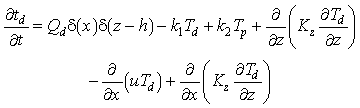(1)(2)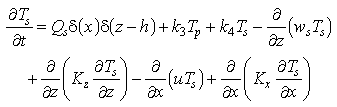(3)

where t is time, x and z are the horizontal and vertical axes, andis the Dirac delta function [e.g., Morse and Feshbach, 1953]. The delta function indicates that Q, Q, and Qare steady point sources located at x = 0 and z = h. The horizontal advection velocity is u and horizontal and vertical turbulent diffusivities are Kand K, respectively. The exchange terms are based on those of Nozaki et al. ; they are independent of particle concentration [Honeyman et al., 1988] because of the reasonably small range of particle concentrations encountered in this particular problem.

Equations (1)-(3) have been solved in a region of constant depth extending from the seafloor to 500 m above. The height was chosen to accommodate a source point centered at 240 m; typical nonbuoyant plumes near the ridge are centered vertically several hundred meters from the seafloor [e.g., Baker and Massoth, 1987]. In the steady state examples to follow, Mn at the source was spread vertically over 75 m, a choice guided by observations. The horizontal extent of the solution region, 330 km westward and 80 km eastward, was chosen to encompass much of the Mn deposition area. The off-axis orientation of the observational transects define the direction of the x axis. The advection velocity, u, consequently represents the off-axis component of mean flow.

The solution is dependent on values given model parameters, examined in detail below, and on model boundary conditions. As do the model equations, the boundary conditions apply only to the hydrothermal component of the Mn field. Background concentrations of Mn are not addressed by the model nor have background Mn concentrations been added to model results.

#### 3.2. Boundary Conditions

At the upstream and downstream ends of the solution domain, for all z and for all three water column phases of Mn, a zero concentration gradient condition,T/dx = 0 (for i = d, p, s), was imposed. This is a numerically tolerant condition, one that allows advective transport of Mn through the upstream and downstream boundaries without having to prescribe the magnitude of Mn concentration or flux there.

At the upper boundary (z = 500 m), for all x and all three Mn phases, a zero vertical flux condition was prescribed: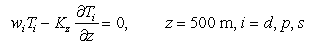(4)

Dissolved Mn has no settling velocity, of course, so w(equation (4)) is zero.

Seafloor boundary conditions must be drawn with care. Consideration must be given to the possibility of remobilization of Mn in the sediment column and subsequent diffusion of dMn back into the water column. Such a "benthic flux" has been argued even for "oxic" sediments at MANOP Site M [Kalhorn and Emerson, 1984; Heggie et al., 1986]. Oxidation of organic matter in the top centimeter of the sediment column is said to result in dMn gradients in pore waters that favor upward diffusion of dMn at the sediment-water interface.

This idea is consistent with the longer held view, based on more coarsely resolved profiles, that Mn is remobilized deeper in the core, diffuses upward, and is reoxidized in the surficial layers of sediments. Cores taken in the vicinity of the JDFR [Jones and Murray, 1985; G. Massoth, unpublished data, 1987] reveal little dMn in the upper few centimeters of the cores compared to that lower in the Mn reducing layer. Remobilization and upward diffusion result in surface sediments enriched in pMn far above that which might be expected of particles settling to the seafloor.

Near-bottom water column gradients of dMn are observed [e.g., Cowen et al., 1986] suggesting diffusion of dMn out of the sediments. The bottom boundary condition for dMn was consequently chosen to permit an upward benthic flux. Diffusive flux of dMn was made proportional to the settling flux of Mn: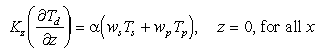(5)

Fixed fraction of the settled Mn is diffused back into the water column as dMn. The proportionality constant,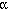, can take a value between 0 and 1. A value of 1 would lead to no Mn accumulation in the sediments and a value of 0 would indicate complete retention of settled Mn in the sediment column and no dMn gradients in the near-bottom water column. An intermediate value needs to be chosen to be consistent with long-term Mn accumulation rates and with dMn water column profiles. While not well defined by measurements, anvalue of 0.1 appears to be consistent with both for the region of study.

The boundary conditions of Mn flux in particulate phases are drawn in analogy to the boundary condition of particle flux at the seafloor: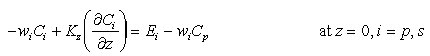(6)

where Eis the sediment erosion rate [e.g., Parthenaides, 1972; Lavelle et al., 1984], and wand Care the settling velocity and particle concentration for settling velocity class i. Total particle flux at the sediment water interface is thus the difference between an upward erosive flux and a downward settling flux.

In analogy, the bottom boundary conditions on fine particulate and macroaggregate Mn were taken to be(7)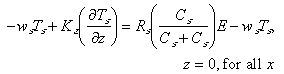(8)

The erosional fluxes have been partitioned so that rates are proportional to the particle concentration of each particle class at the seafloor. The erosion terms are also proportional to Rand R, the mass fractions of Mn on fine and macroaggregate particles. In the water column, these fractions are equal to the functions T/Cand T/C, respectively, evaluated at a point of interest. At the sediment-water interface Rand Rmay exceed lower water column values because diagenetic processes can enrich sedimented particles with Mn. For the purposes of these calculations, however, R= T/Cand R= T/Care based on values of component concentrations just above the seafloor. Under these assumptions, equations (7) and (8) result in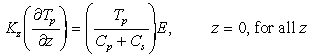(9)(10)

Manganese fluxes at the seafloor (equations (5), (7), and (8)), when summed, yield the net Mn accumulation rate, D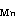in the sediments: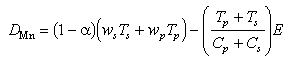(11)

This net Mn accumulation rate is seen to be the sum of the settling flux in both particulate phases less the sum of dissolved benthic and particle erosional fluxes.

Specifying the boundary conditions (equations (4), (5), (9), and (10)) allows equations (1)-(3) to be integrated. Steady state and time dependent solutions were obtained numerically; the steady solution was achieved by integrating in time until no further changes in variable fields occurred. Equations (1)-(3) were center differenced to second-order accuracy in both space dimensions and implicitly forward differenced in time. The Tvalues were evaluated on grids staggered with respect to the u and Kgrids. Final grid intervals were 5000 m in the x direction and 25 m and 6.25 m in the z direction for steady and time dependent problems, respectively. Kwas reduced to its final value (Table 2) during approach to steady state. Plume thicknesses were sensitive to the value of K(0.8 versus 2.0 cm/s). For the time dependent solution, the vertical advection term was upstream differenced and the time step judiciously chosen (dt =z/w) to curtail unwanted numerical diffusion.

The source strength, Q, was adjusted so that the dMn and pMn would concurrently have magnitudes comparable to those observed in the transects (Figures 1a-1b ); Qand Qare both zero for hydrothermal Mn. The horizontal advection velocity, u, and macroaggregate scavenging rate, k, were adjusted so that measured and modeled distributions of Mn accumulating in sediments would be similar.